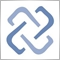# Discussion of article "Probability theory and mathematical statistics with examples (part I): Fundamentals and elementary theory"Moderator
226723

New article Probability theory and mathematical statistics with examples (part I): Fundamentals and elementary theory has been published:

Trading is always about making decisions in the face of uncertainty. This means that the results of the decisions are not quite obvious at the time these decisions are made. This entails the importance of theoretical approaches to the construction of mathematical models allowing us to describe such cases in meaningful manner.

I would like to highlight two such approaches: probability theory and game theory. Sometimes, they are combined together as the theory of "games against nature" in topics related to probabilistic methods. This clearly showcases the existence of two different types of uncertainty. The first one (probabilistic) is usually associated with natural phenomena. The second one (purely game-related) is associated with the activities of other subjects (individuals or communities). The game uncertainty is much more difficult to deal with theoretically. Sometimes, these uncertainties are even called "bad" and "good". The progress in understanding of initially game-related uncertainty is often associated with reducing it to a probabilistic form.

In case of financial markets, the uncertainty of the game nature is obviously more important, since the activity of people is the key factor here. The transition to probabilistic models here is usually based on considerations of a large number of players, each of whom individually has little effect on price changes. In part, this is similar to the approach used in statistical physics, which led to the emergence of a scientific approach called econophysics.

In fact, the topic of such a transition is very interesting, non-trivial, and deserves more detailed consideration. Hopefully, the related articles will appear on our forum someday. In this article, we will look at the very foundations of probability theory and mathematical statistics.

Author: Aleksey Nikolayev